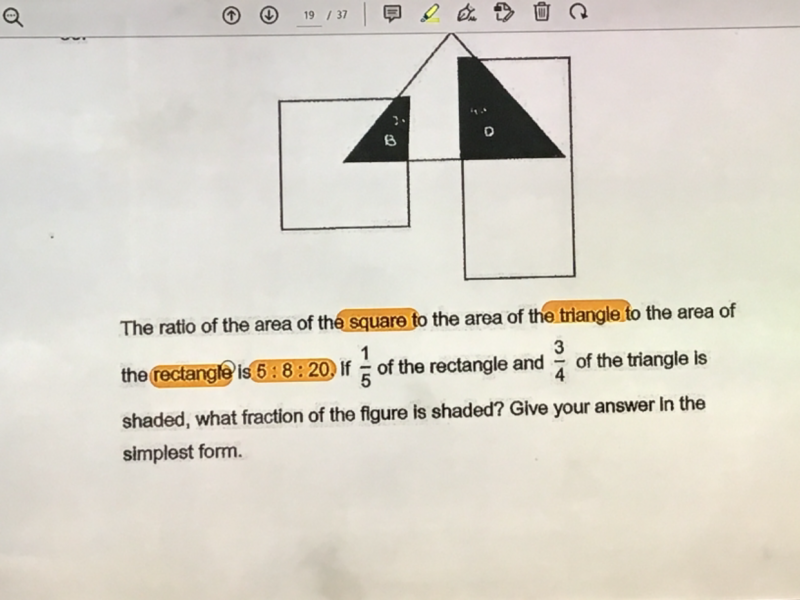# QuestionPls help to solve, thx

Square         Triangle         Rectangle          Total

5u                8u                    20u                   33u

1/5 x 20u = 4u  = right shaded part of triangle

Total shaded part of triangle = 3/4 x 8u = 6u     =>     Unshaded part = 8u-6u = 2u

Hence total figure taking away the overlapping parts = 33u – 6u = 27u   or  5u+2u+20u = 27u

Fraction of shaded part of the figure = Shaded part of triangle / total figure

=  6u/27u = 2/9

0 Replies 1 Like